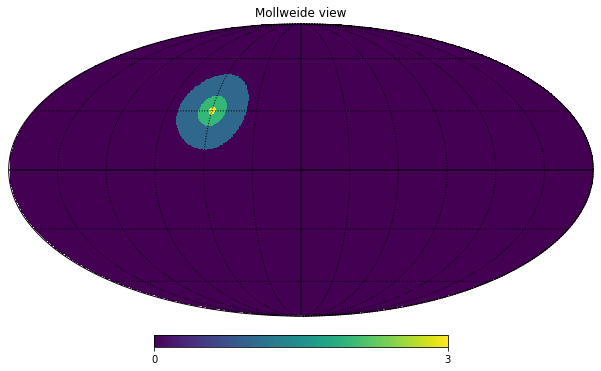import healpy as hp
import numpy as np


lonlat=True switches ang2vec from requiring colatitude $\theta$ and longitude $\phi$ in radians to longitude and latitude in degrees (notice that also the order changes)

lon = 60
lat = 30
vec = hp.ang2vec(lon, lat, lonlat=True)

nside = 256


query_disc returns a list of pixels, by default in RING ordering, let's check their length:

list(map(len, [large_disc, small_disc, tiny_disc]))

[23715, 3829, 237]

## Create a map and plot it in Mollweide projection

m = np.zeros(hp.nside2npix(nside))

m[large_disc] = 1
m[small_disc] = 2
m[tiny_disc] = 3

hp.mollview(m)
hp.graticule()

0.0 180.0 -180.0 180.0
The interval between parallels is 30 deg -0.00'.
The interval between meridians is 30 deg -0.00'.